Cost function, back propagation, forward propagation, unrolling parameters, gradient checking, and random initialization.

## 1. Cost Function and Back Propagation

I would like to give full credits to the respective authors as these are my personal python notebooks taken from deep learning courses from Andrew Ng, Data School and Udemy :) This is a simple python notebook hosted generously through Github Pages that is on my main personal notes repository on https://github.com/ritchieng/ritchieng.github.io. They are meant for my personal review but I have open-source my repository of personal notes as a lot of people found it useful.

### 1a. Cost Function

• Neural Network Introduction
• One of the most powerful learning algorithms
• Learning algorithm for fitting the derived parameters given a training set
• Neural Network Classification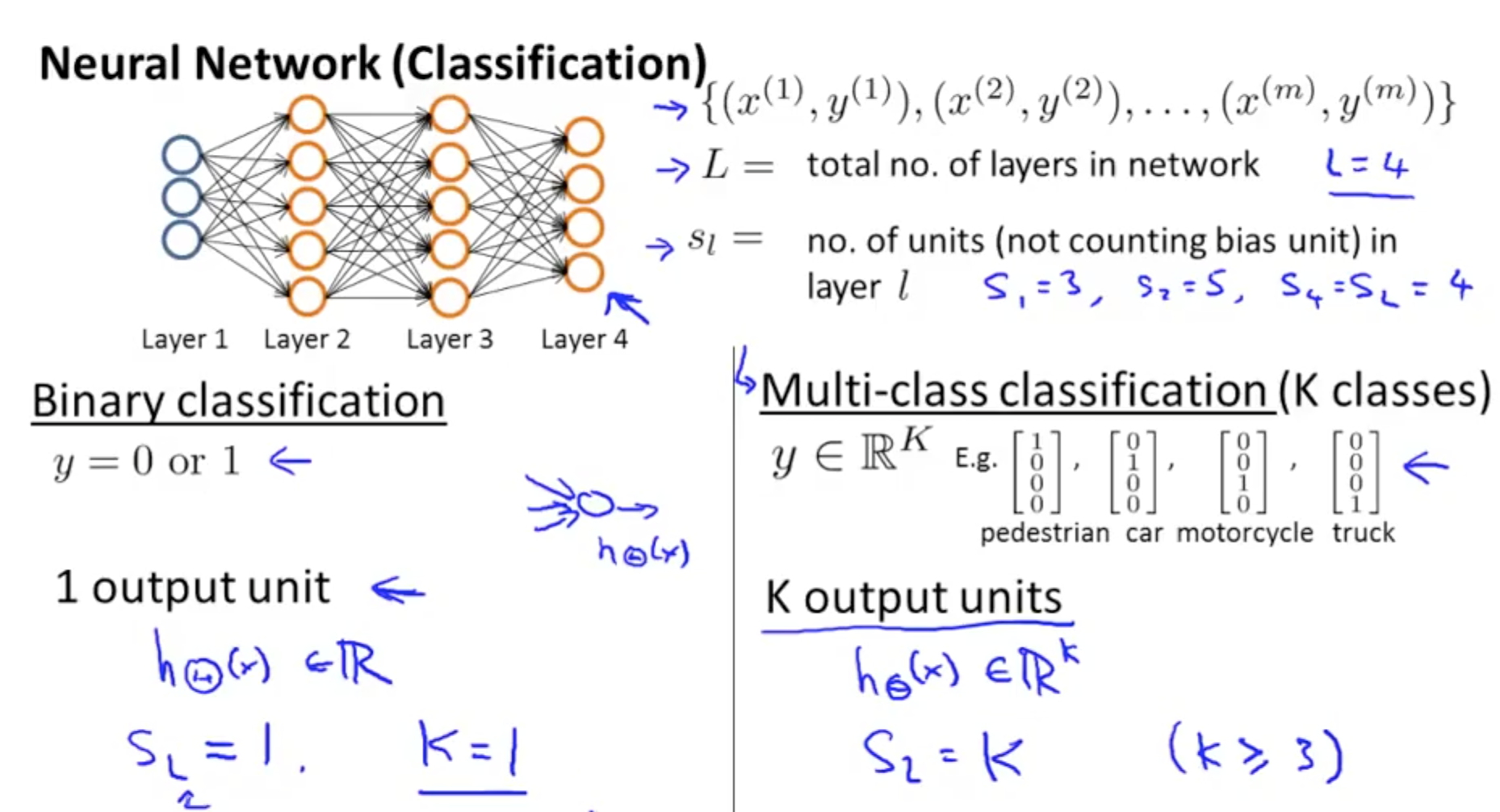• Cost Function for Neural Network
• Two parts in the NN’s cost function
• First half (-1 / m part)
• For each training data (1 to m)
• Sum each position in the output vector (1 to K)
• Second half (lambda / 2m part)
• Weight decay term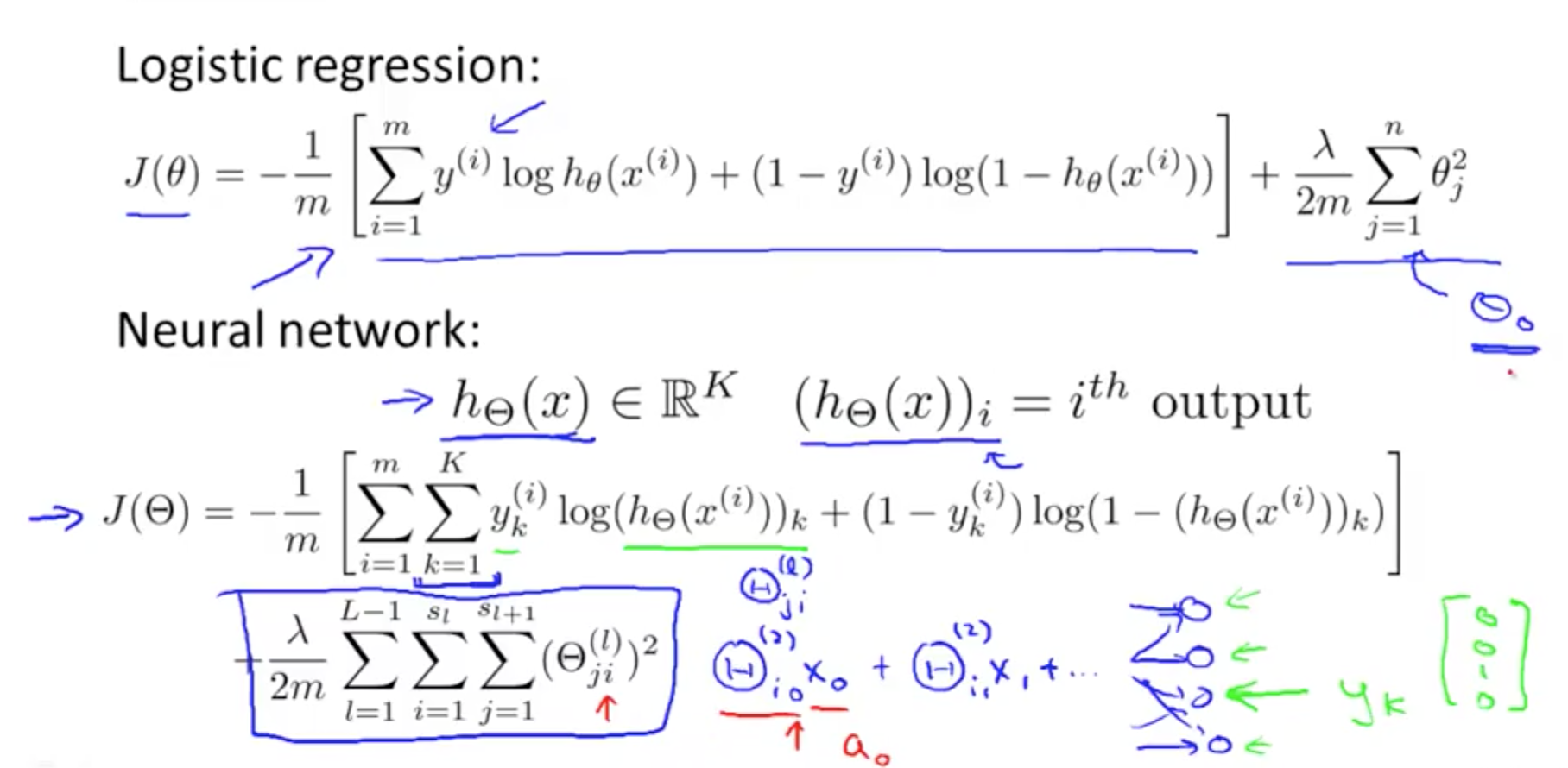### 1b. Overview

• Forward propagation
• Algorithm that takes your neural network and the initial input (x) and pushes the input through the network
• Back propagation
• Takes output from your neural network H(Ɵ)
• Compares it to actual output y
• Calculates H(Ɵ)’s deviation from actual output
• Takes the error H(Ɵ) - y from layer L
• Back calculates error associated with each unit from the preceding layer L - 1
• Error calculated from each unit used to calculate partial derivatives
• Use partial derivatives with gradient descent to minimise cost function J(Ɵ)
• Basic things to note
• Ɵ matrix for each layer in the network
• This has each node in layer l as one dimension and each node in l+1 as the other dimension
• Δ matrix for each layer
• This has each node as one dimension and each training data example as the other

### 1c. Backpropagation Algorithm

• Purpose is to find parameters Ɵ that minimizes J(Ɵ)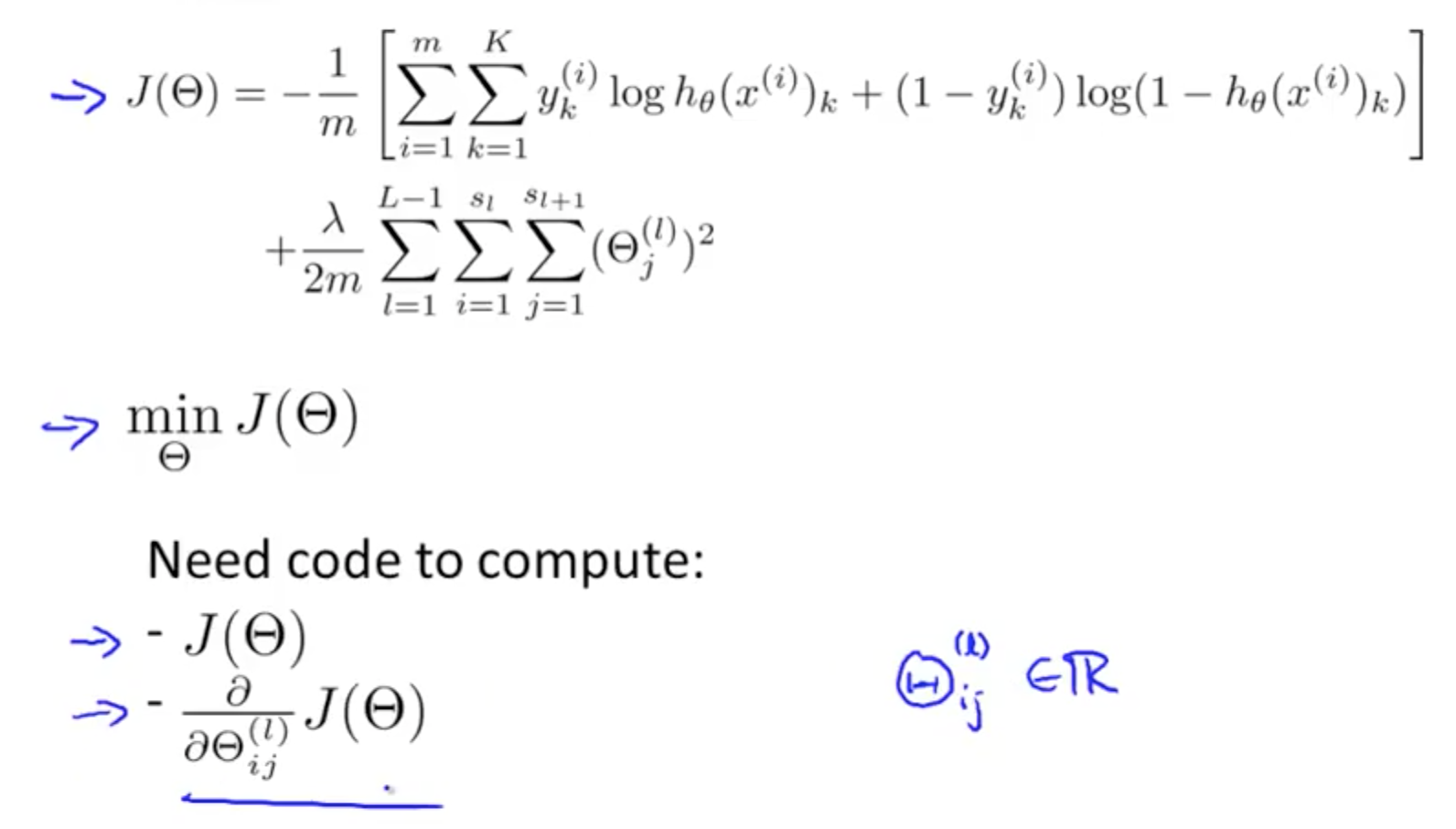• Forward Propagation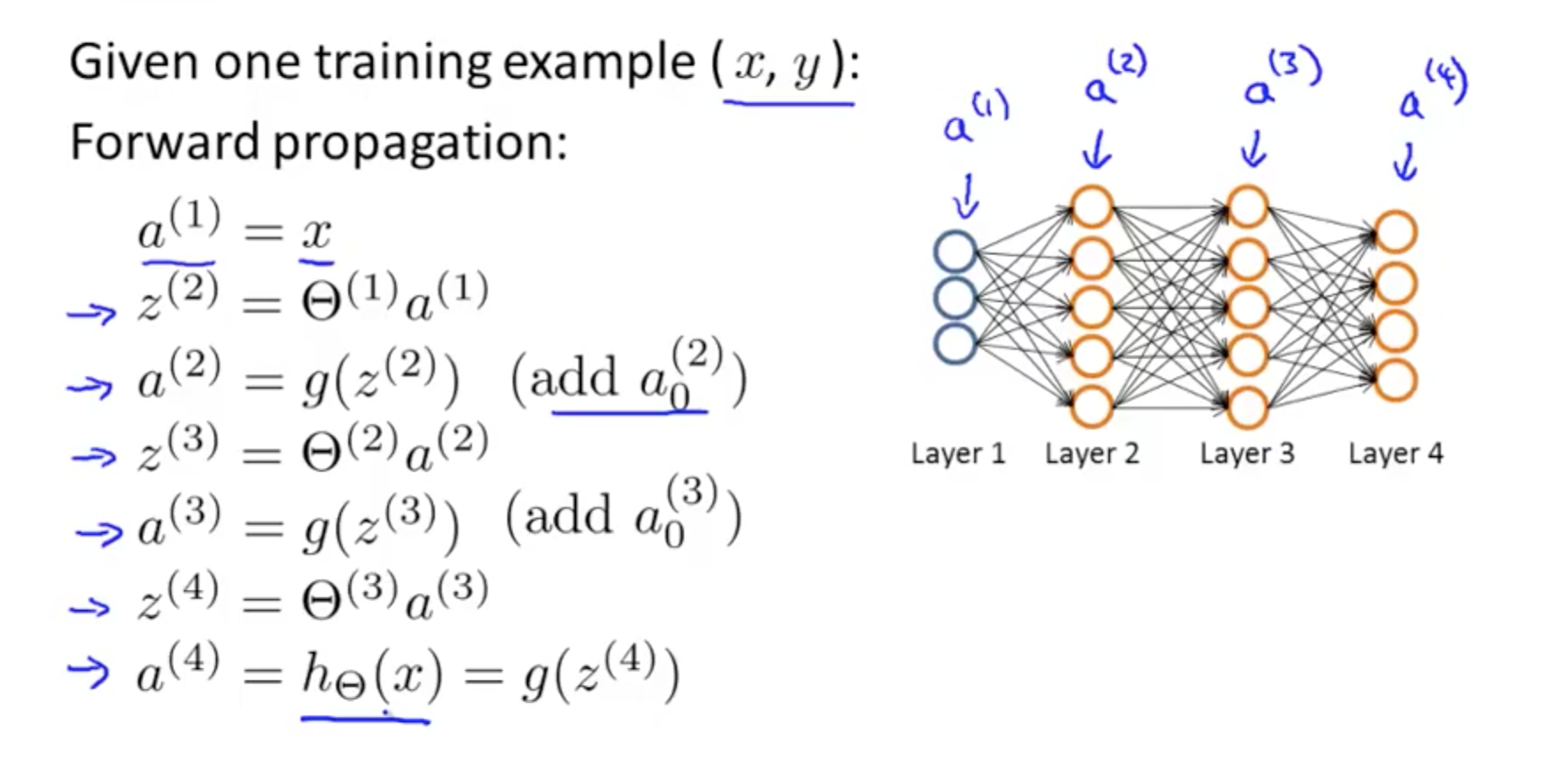• Backpropagation Equation
• For each node we can calculate δj^l
• This is the error of node j in layer l
• aj^l is the activation of node j in layer l
• activation would have some error compared to the “real” value
• delta calculates this error
• But the “real” value is an issue
• The neural network is an artificial construct we made
• We have to use the actual output, y and work from there
• Work from the last/output layer, L
• If L = 4
• delta^4 = a^4 - y
• We can determine error in the preceding layers once we’ve the error from the output layer
• delta^3
• delta^2
• There is no error in the first layer as it’s simply the actual input x
• Once we get the deltas, we can calculate the gradient or the partial derivative of the cost function
• Terms in equation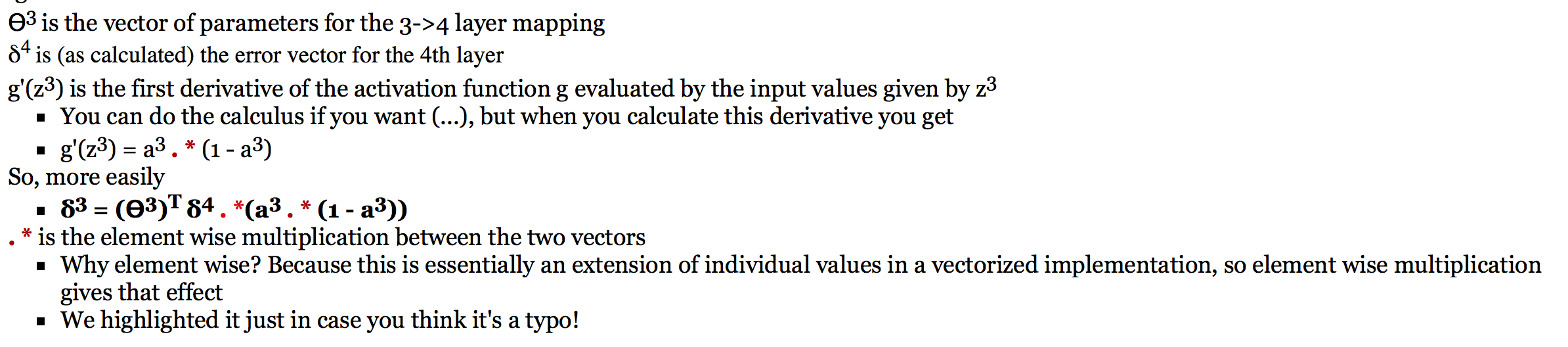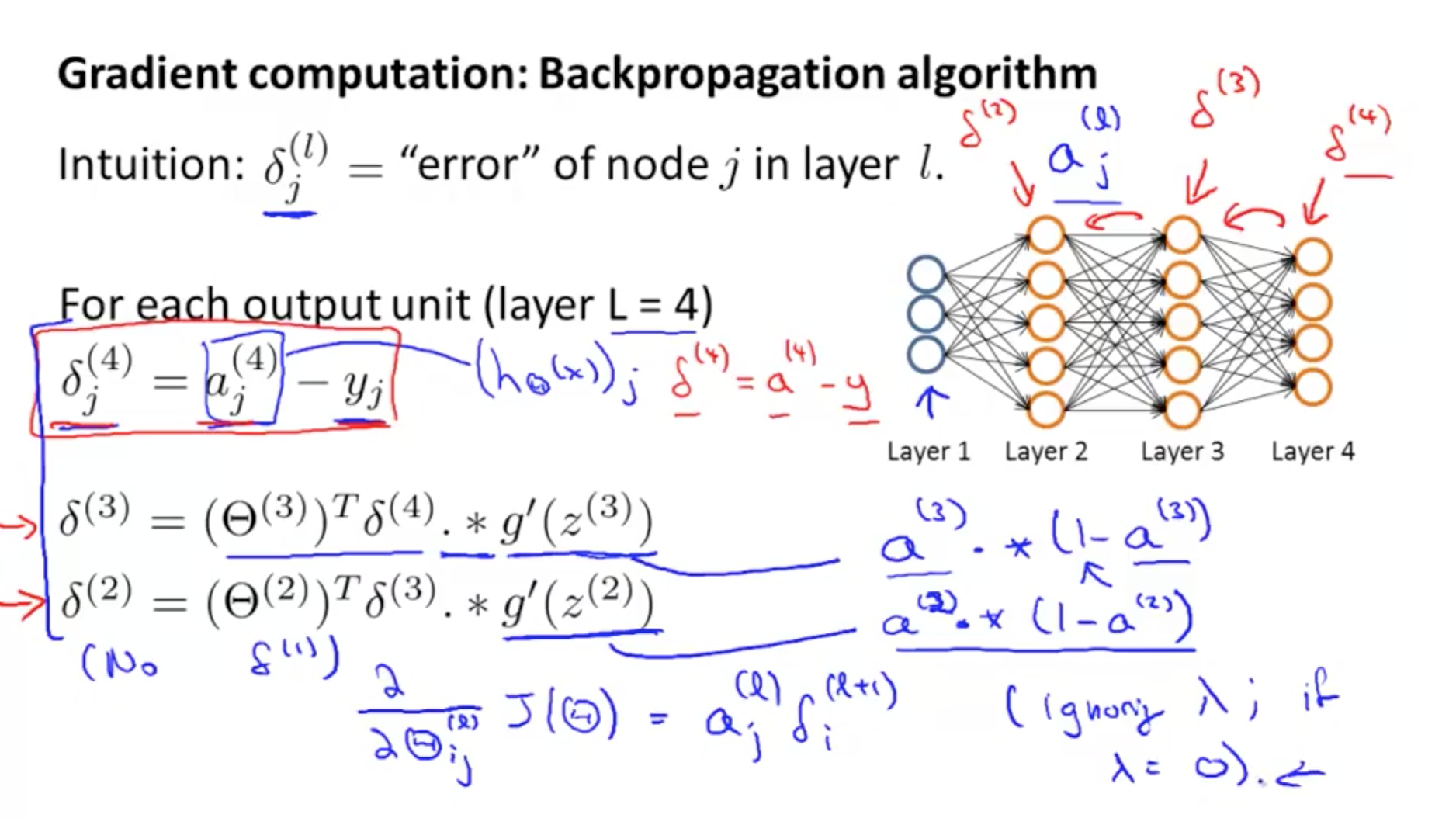• Dimensions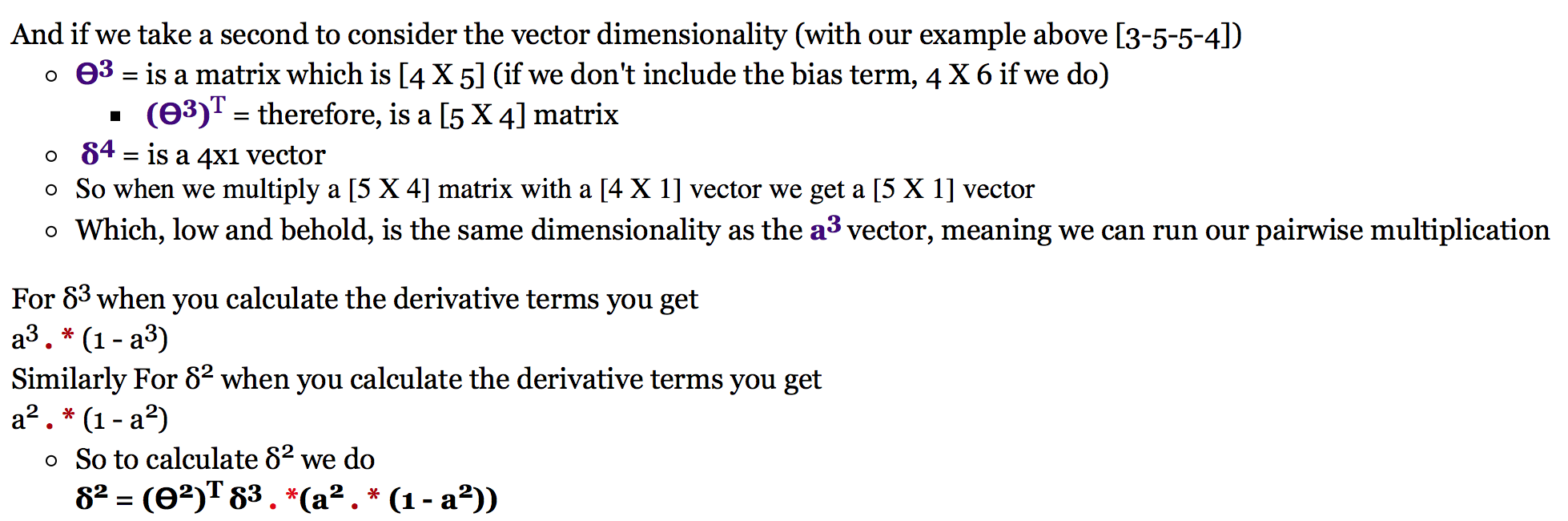• Backpropagation Algorithm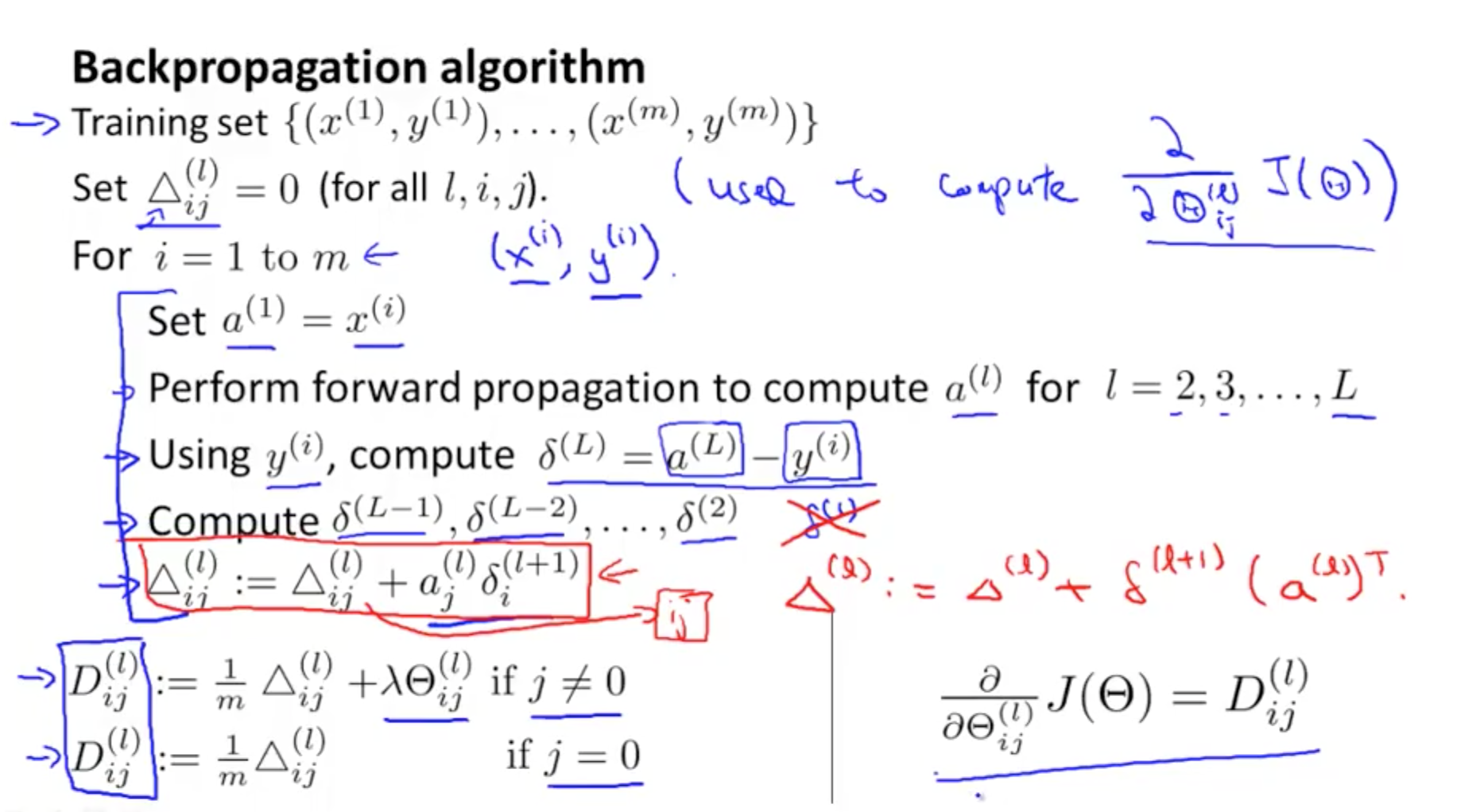### 1d. Backpropagation Intuition

• Forward Propagation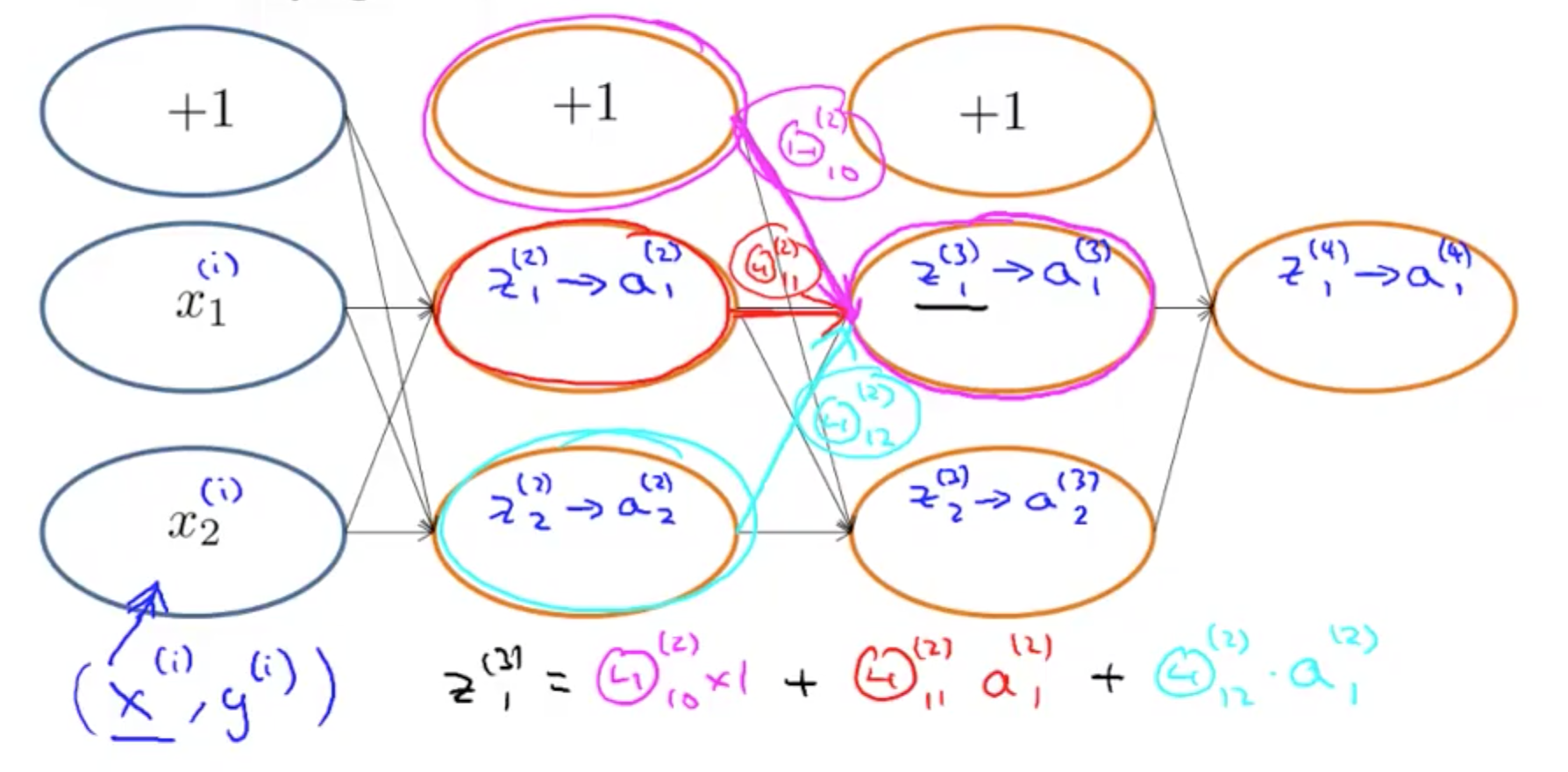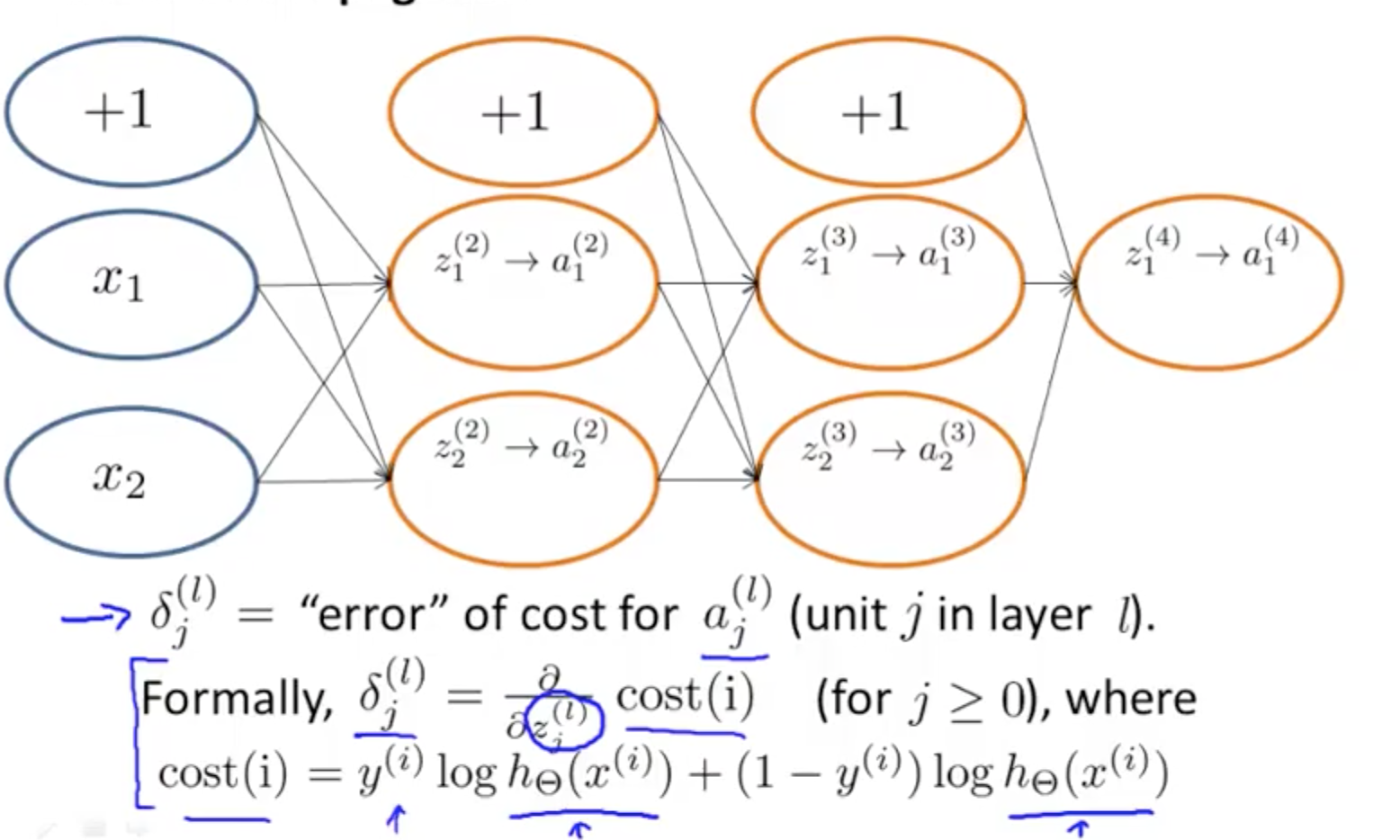• Backpropagation’s cost function with 1 output unit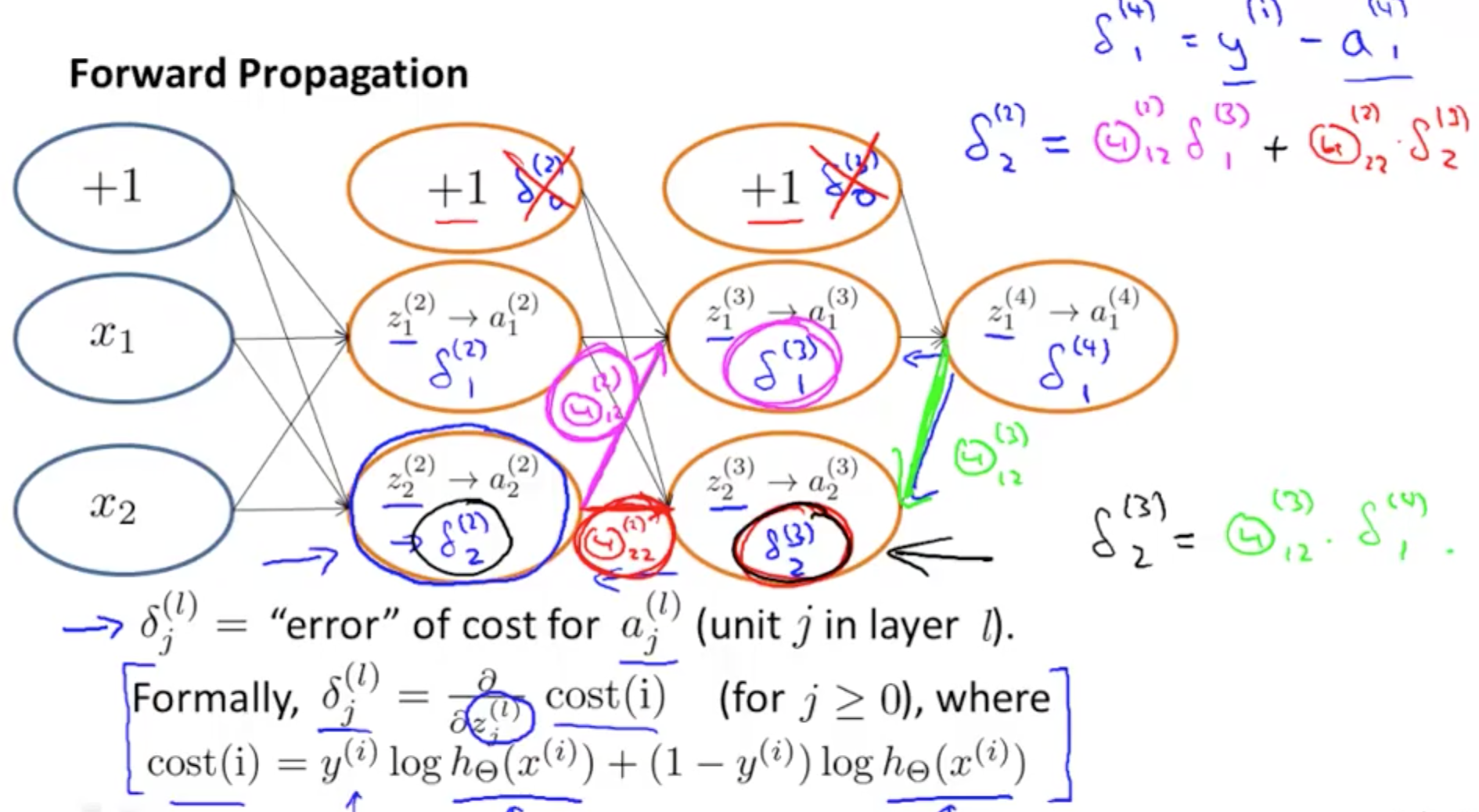## 2. Backpropagation in Practice

### 2a. Unrolling Parameters

• Issue here is that we’ve to unroll the matrices into vectors for the algorithm fminunc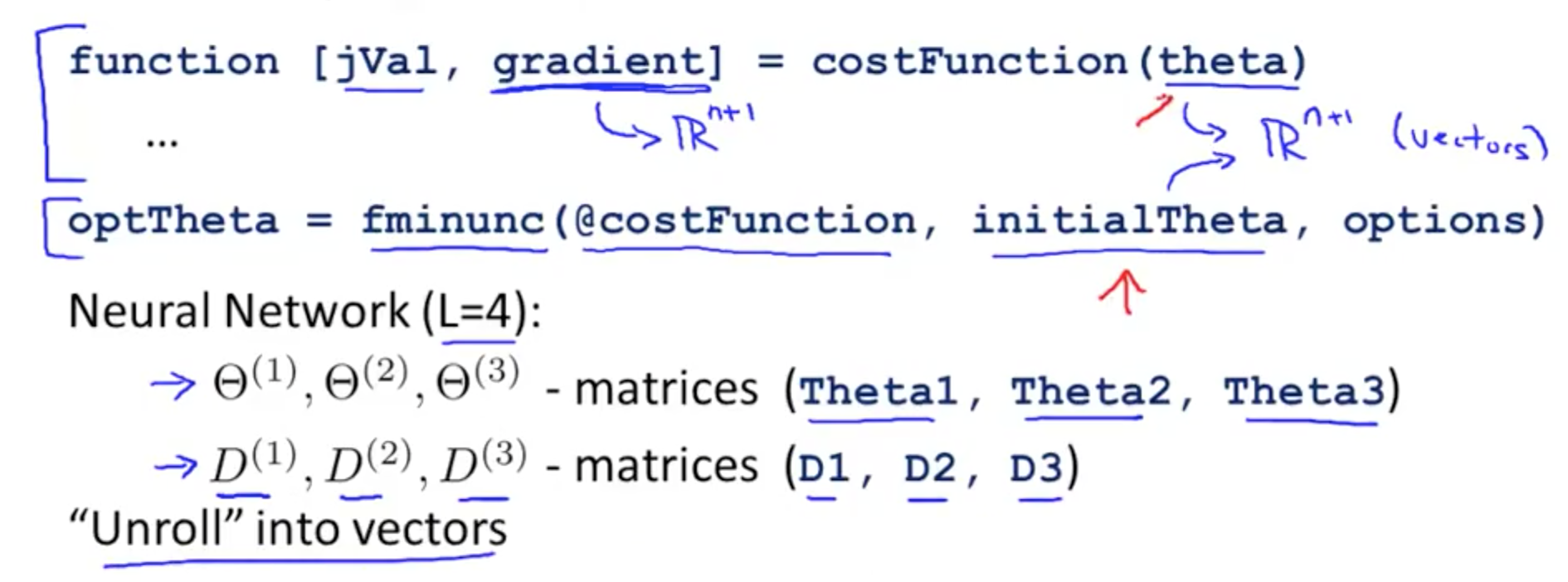• Example
• s1 (layer 1 units) = 10
• s2 (layer 2 units) = 10
• s3 (layer 3 units) = 1
• Ɵ1(:) unrolls into a vector
• You can go back with reshape by pulling up each bunch of elements and reshape accordingly
• thetaVec(1:110) pulls up Ɵ1, the first 10 x 11 elements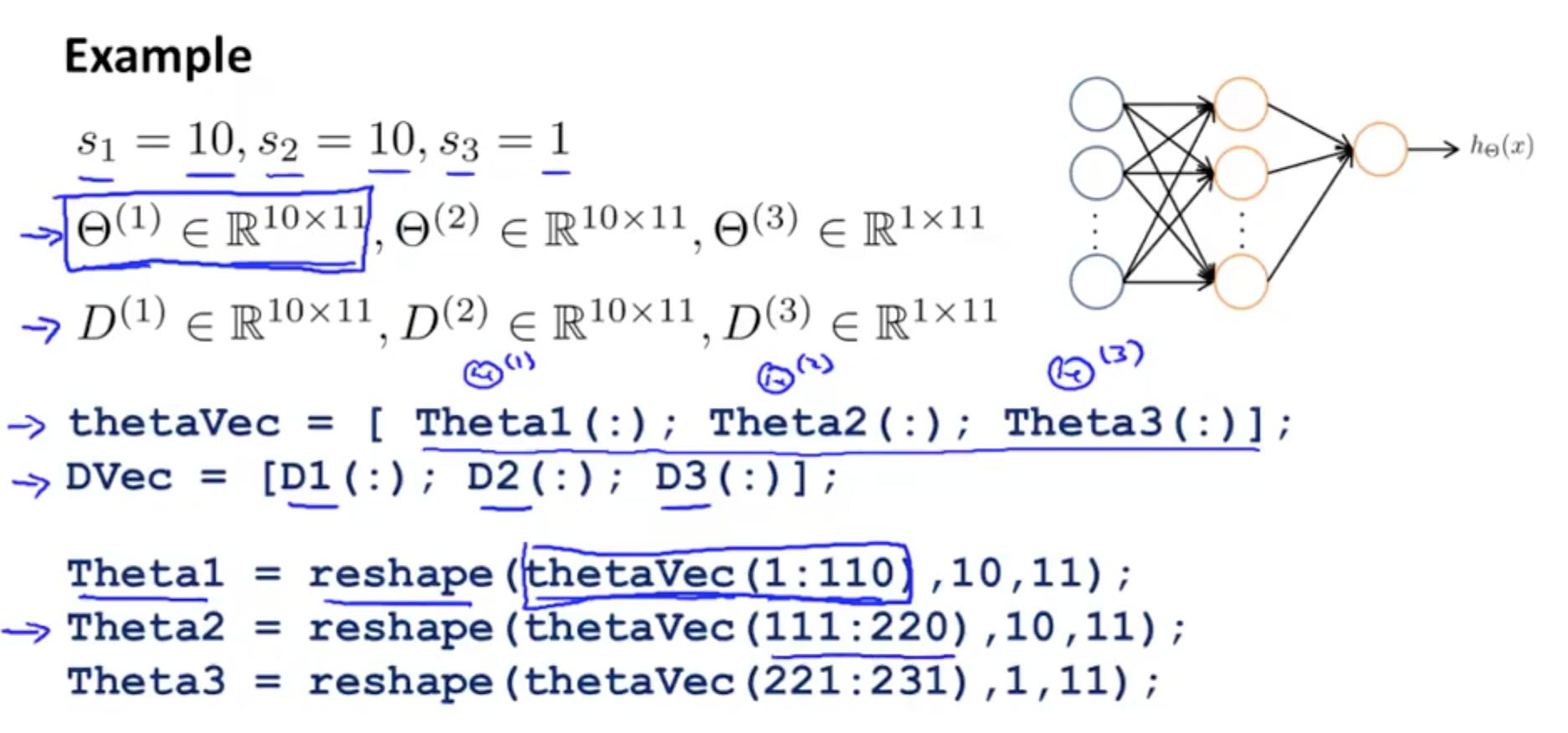• Learning Algorithm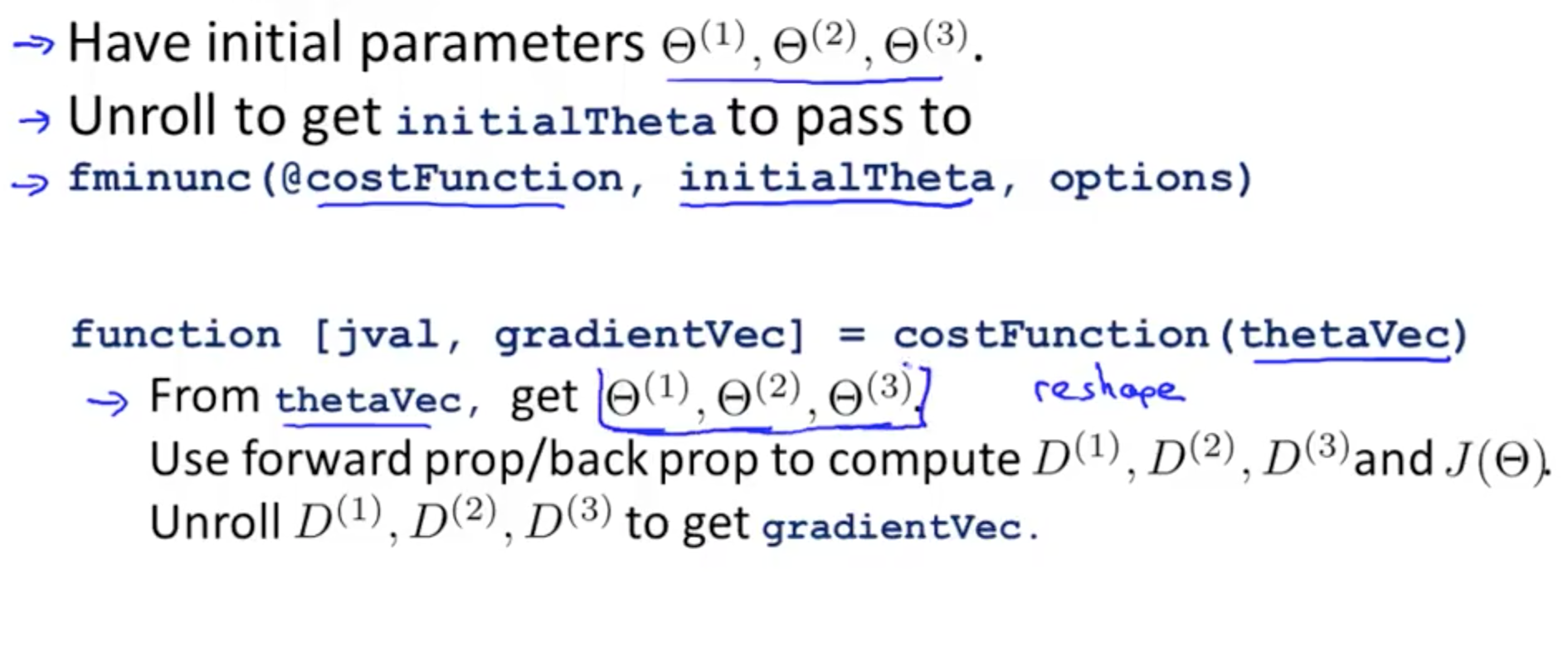• It might look like J(Ɵ) is decreasing
• But you might not know that there is a bug
• You can do gradient checking to ensure your implementation is 100% correct
• We can numerically estimate gradients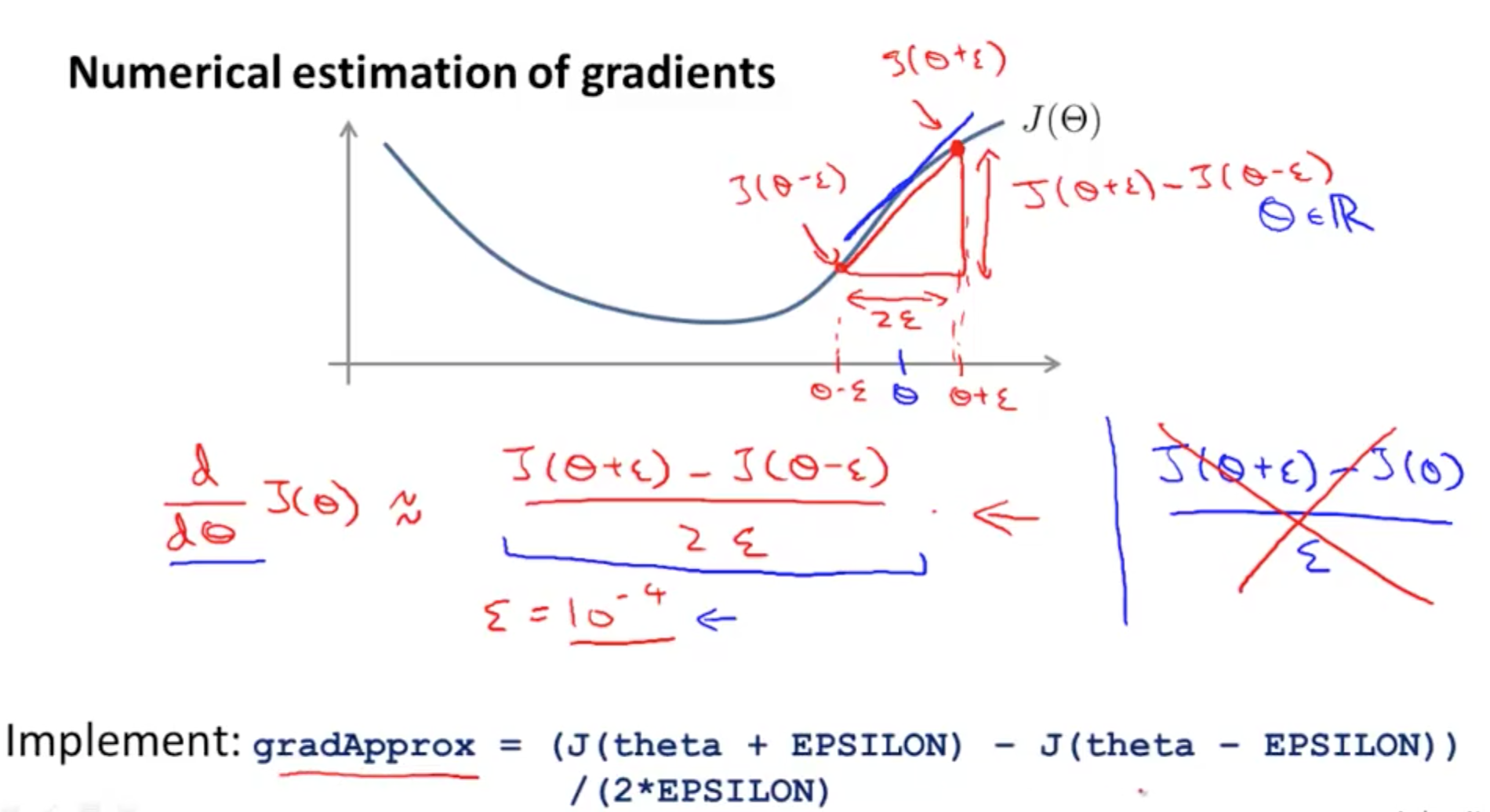• Octave implementation
• n is the dimension of Ɵ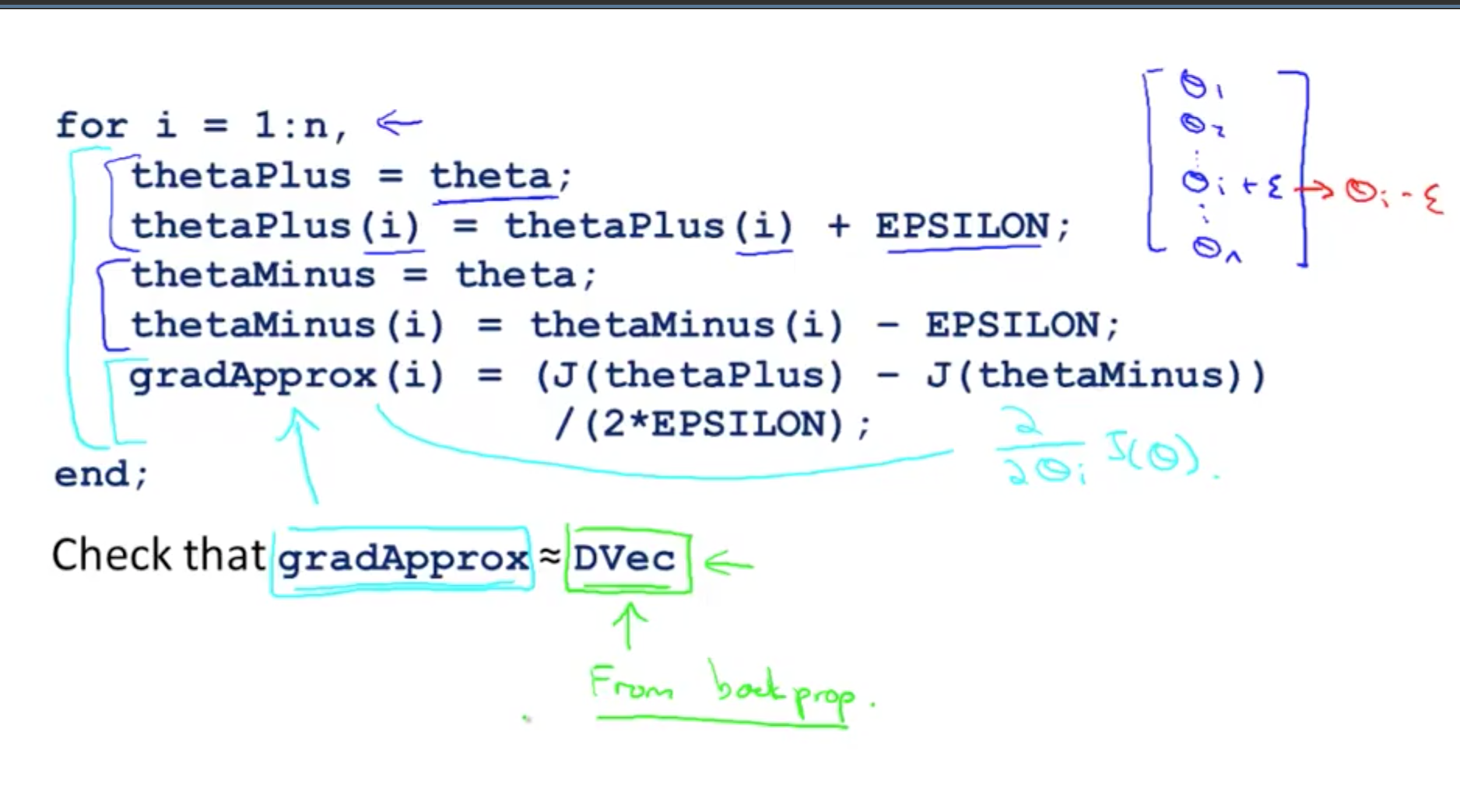• Implementation Note
• Always turn off your numerical checking code as it’s very slow to execute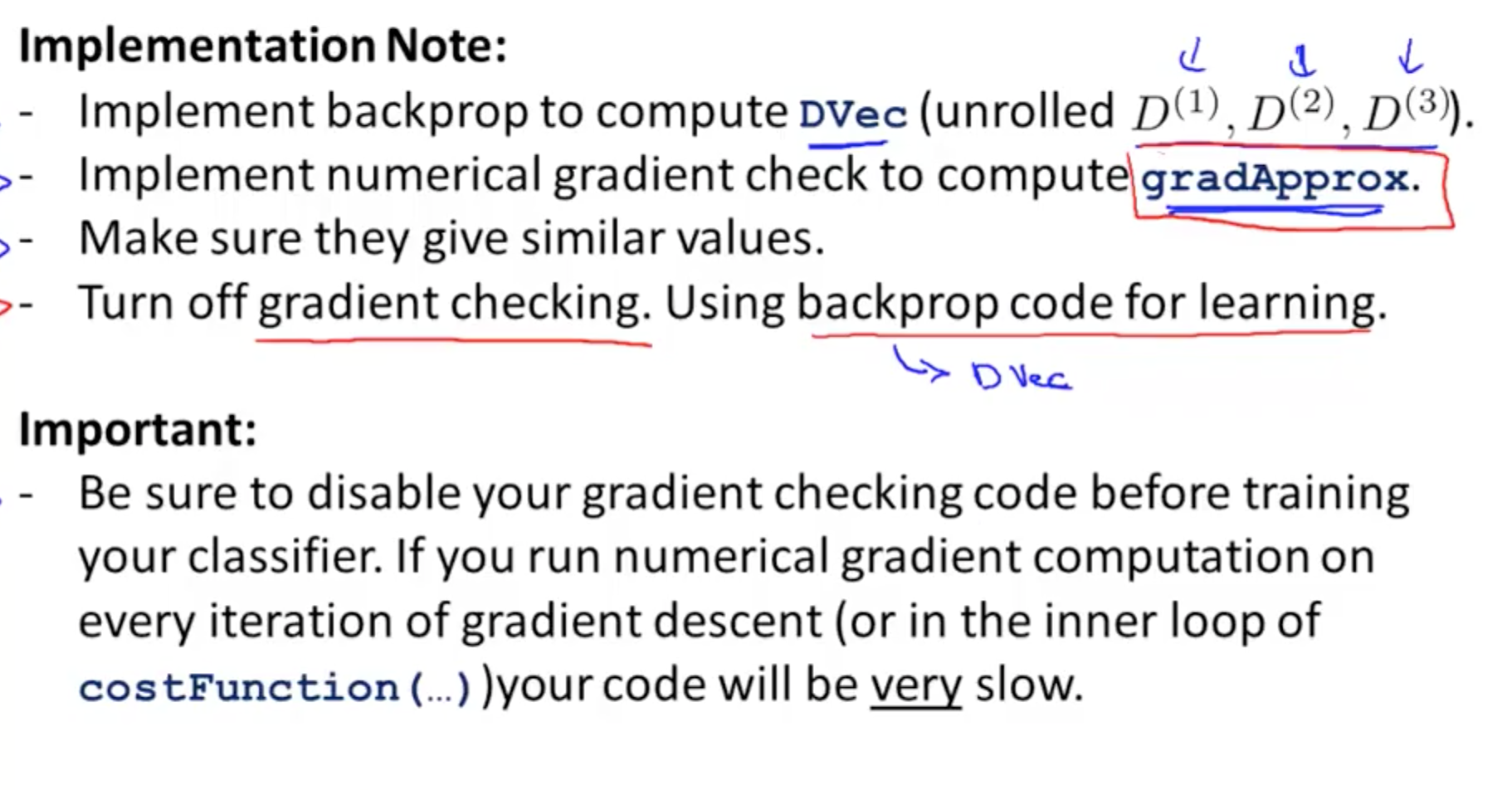### 2c. Random Initialization

• Initial value of Ɵ
• Initialising Ɵ with zeros would not work for neural networks
• After each update, parameters corresponding to inputs going into each of two hidden units are identical
• You NN would not learn anything interesting
• Solution is to have a random initialisation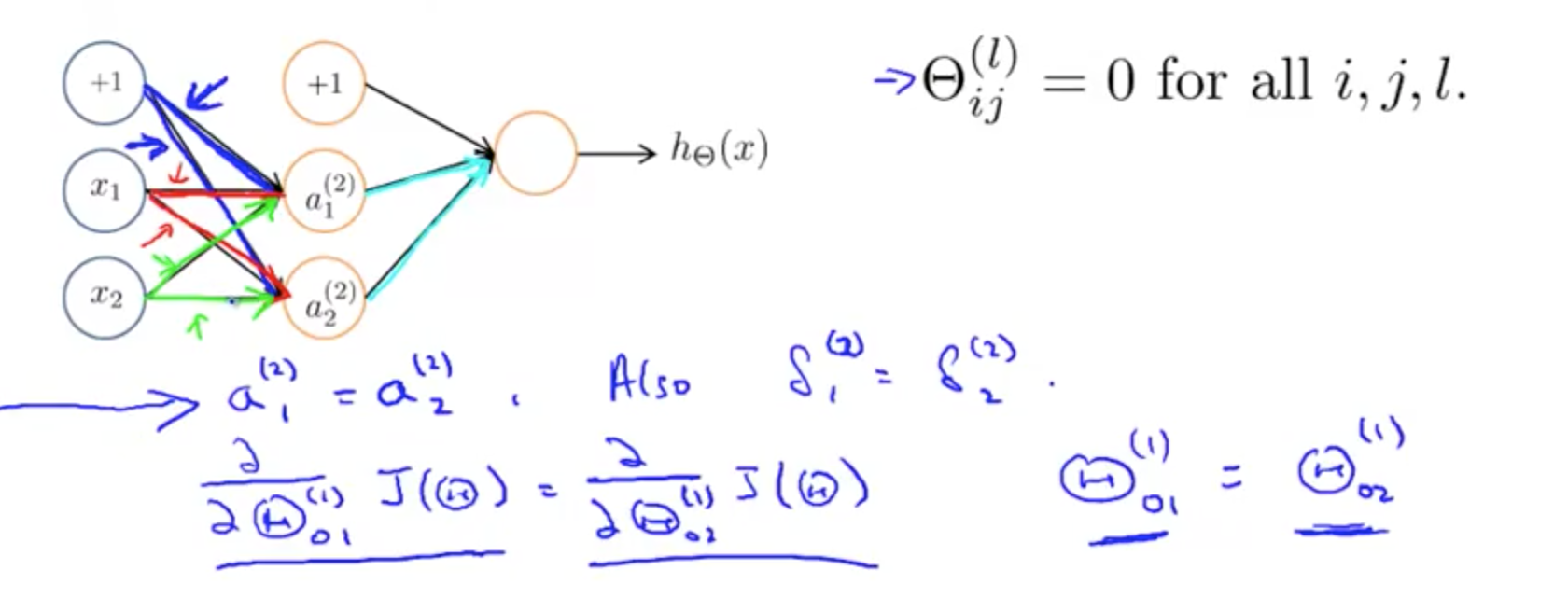• Random initialization: symmetry breaking
• You will be able to use gradient descent or any advanced optimisation methods to find good values for theta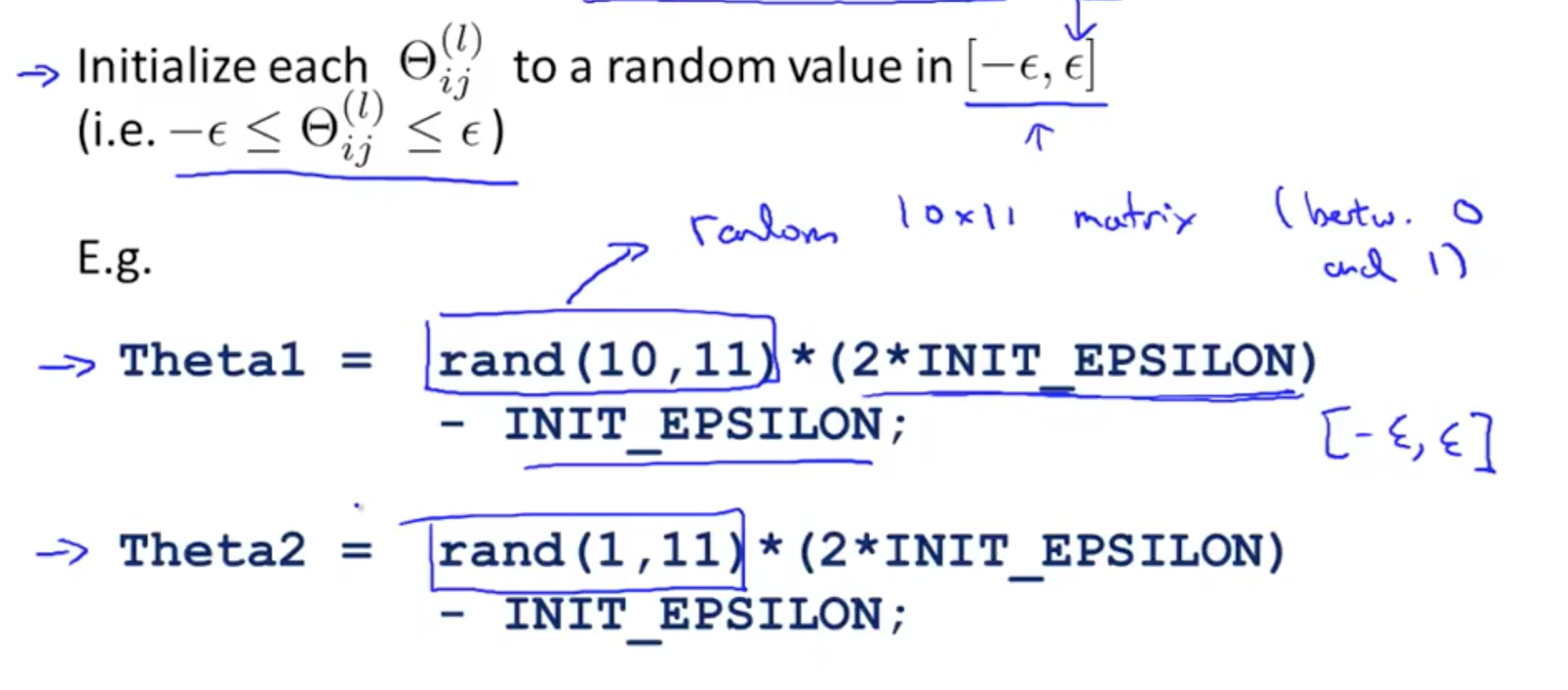### 2d. Putting everything together

• NN’s layers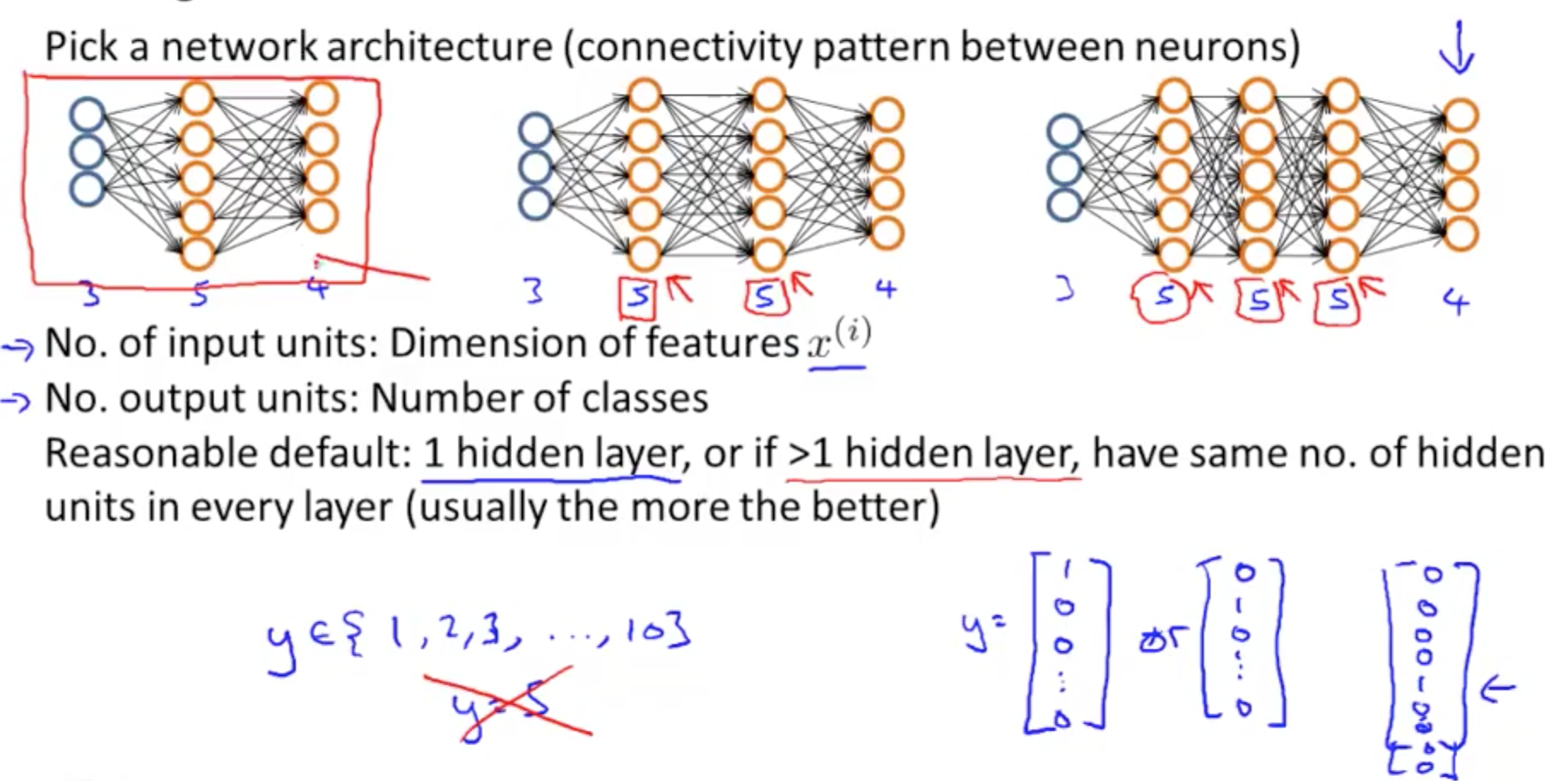• Six steps for training a neural network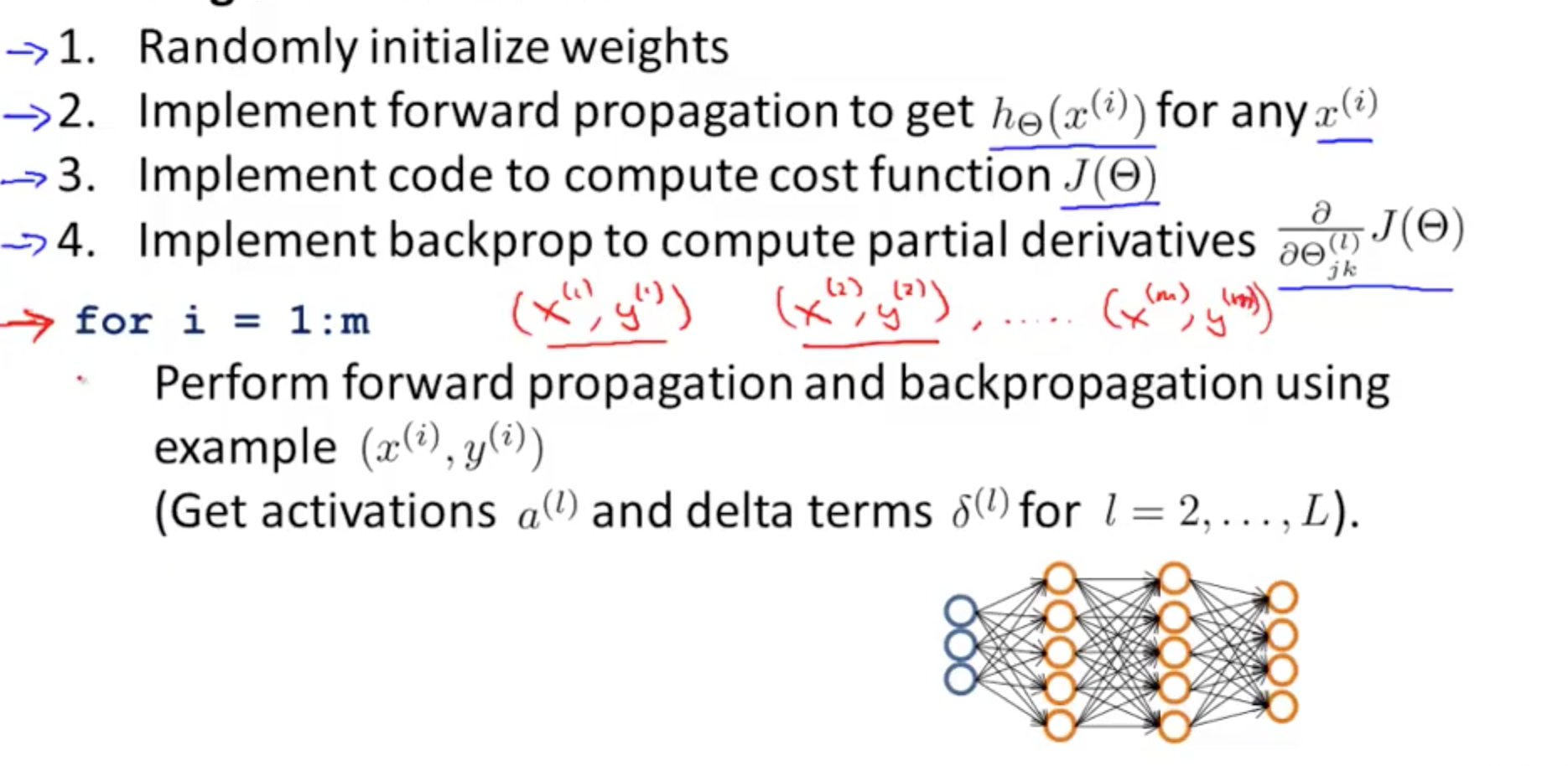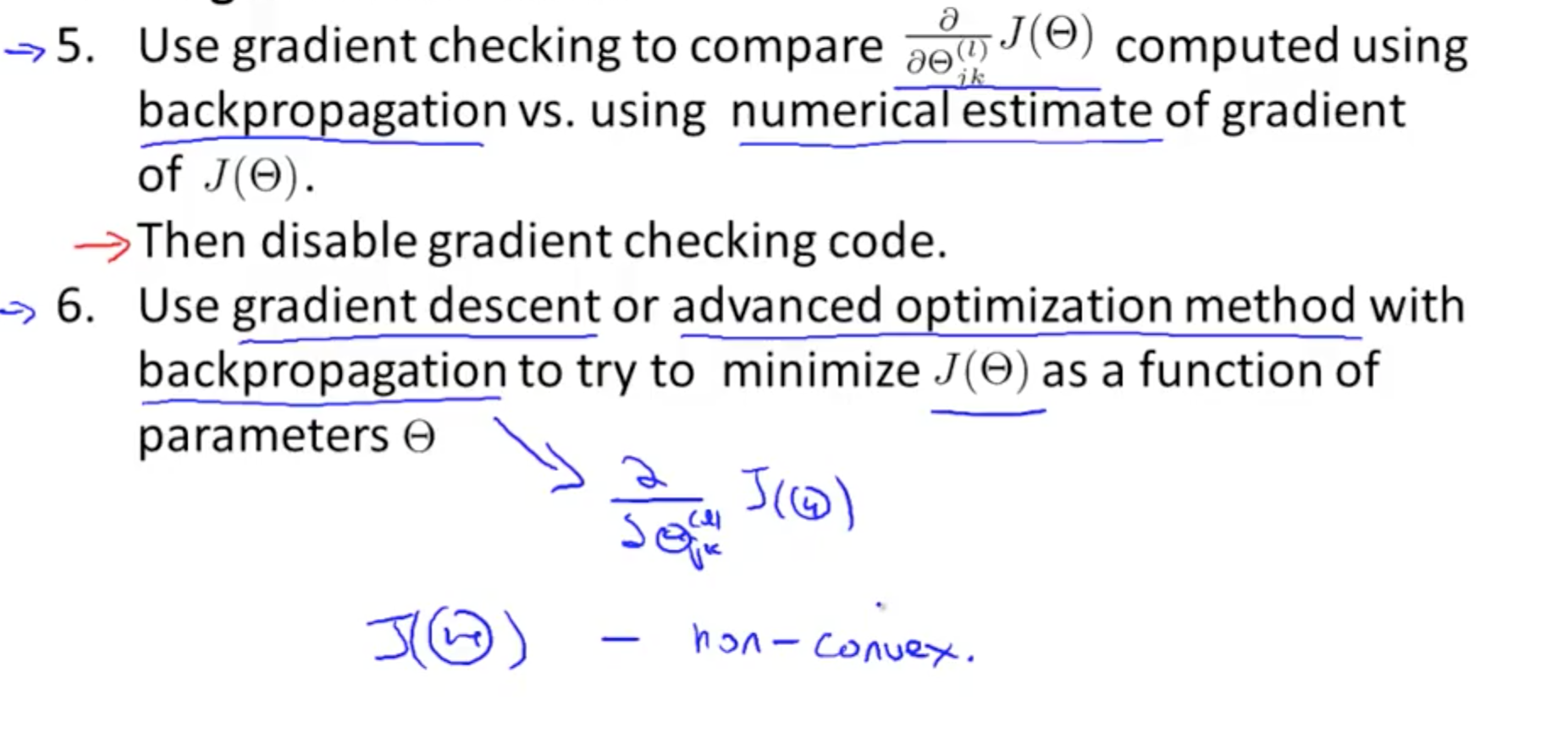• J(Ɵ) closeness to actual values
• Gradient descent: taking little steps downhill to find lowest J(theta)
• Backpropagation: computing direction of gradient
• Able to fit non-linear functions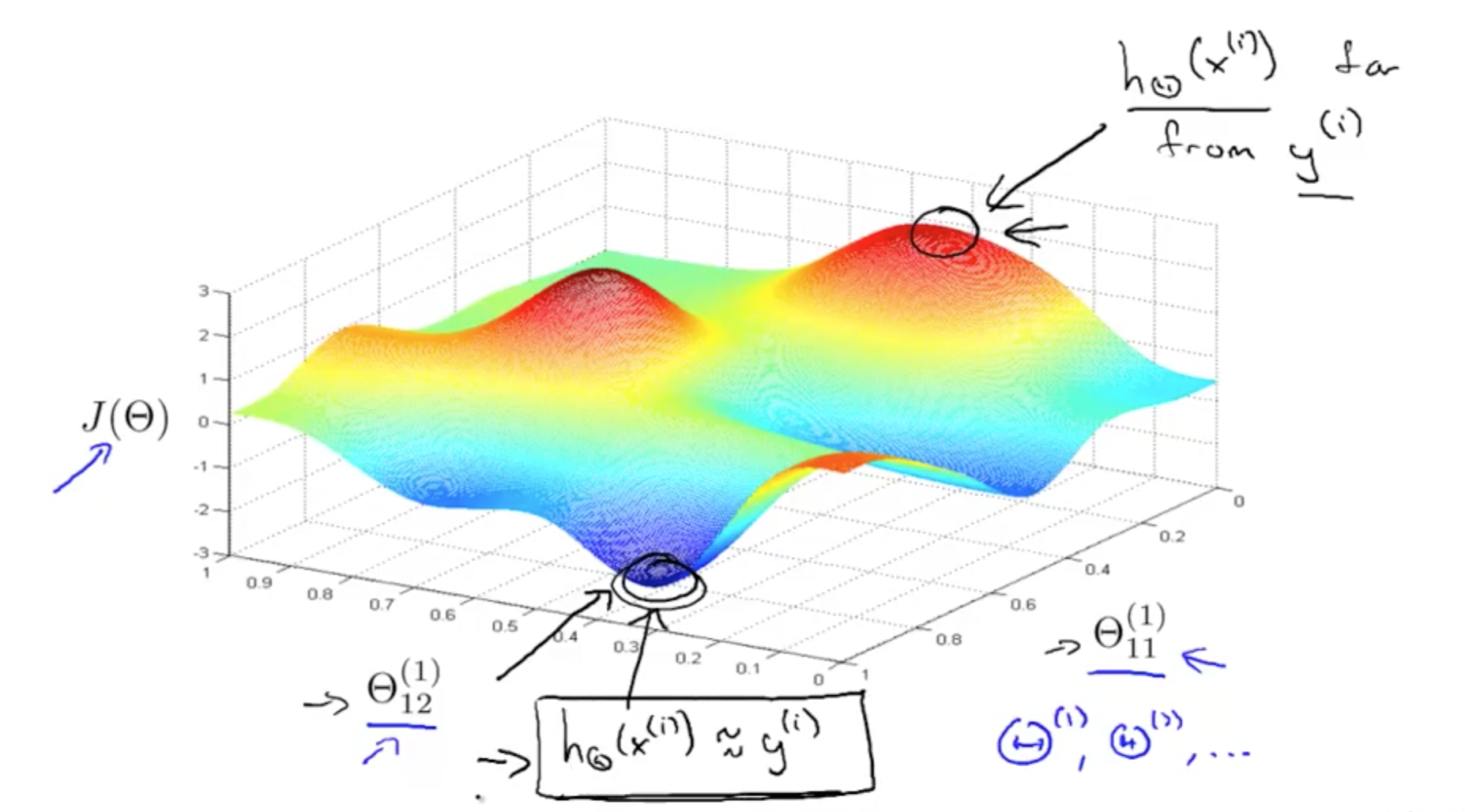Tags: